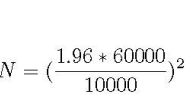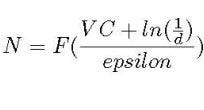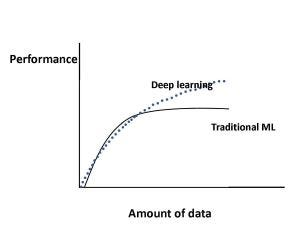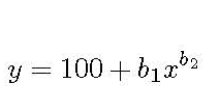• Machine Learning
• Theophano Mitsa
• MAY 10, 2019

# How Do You Know You Have Enough Training Data?

There is some debate recently as to whether data is the new oil  or not . Whatever the case, acquiring training data for our machine learning work can be expensive (in man-hours, licensing fees, equipment run time, etc.). Thus, a crucial issue in machine learning projects is to determine how much training data is needed to achieve a specific performance goal (i.e., classifier accuracy). In this post, we will do a quick but broad in scope review of empirical and research literature results, regarding training data size, in areas ranging from regression analysis to deep learning. The training data size issue is also known in the literature as sample complexity. Specifically, we will:

• Present empirical training data size limits for regression and computer vision tasks.
• Given the desired power of a statistical test, discuss how to determine sample size. This is a statistic topic; however, given its closeness to the determination of training data size in machine learning, it is appropriate to include it in this discussion.
• Present results from statistical theory learning, regarding what controls the size of training data.
• Provide an answer to the question: As training data grows, will performance continue to improve? What happens in the case of deep learning?
• We will present a methodology to determine training data size in classification.
• Finally, we will provide an answer to the question: Is the growth of training data, the best way to deal with imbalanced data?

### Empirical Bounds for Training Data Size

Let us first discuss some widely used empirical ways to determine the size of the training data, according to the type of model we use:

· Regression Analysis: According to the 1 in 10 rule of thumb, we need 10 cases per predictor. Different versions of this, such as 1 in 20 to address regression coefficient shrinkage, are discussed in . One exciting, recently developed, variation for binary logistic regression is presented in . Specifically, the authors estimate training data size by taking into consideration the number of predictor variables, total sample size, and the fraction of positive samples/total sample size.

• Computer Vision: For image classification using deep learning, a rule of thumb is 1,000 images per class, where this number can go down significantly if one uses pre-trained models .

### Hypothesis Testing Sample Size Determination

Hypothesis testing is one of the tools that a data scientist can use to test the difference between populations, determine the effect of a new drug, etc. Here it is quite often desirable to determine the sample size, given the power of the test.

Let us consider this example: A tech giant has moved to city A, and house prices there have increased dramatically. A reporter wants to find out, what is the new average price for condos. How many condo sale prices should he average, having a 95% confidence, given the standard deviation of condo prices at 60K and the acceptable margin of error at 10K? The corresponding formula is shown below; N is the sample size he will need, 1.96 is the number from the standard normal distribution corresponding to 95% confidenceEstimation of sample size

According to the above equation, the reporter will need to consider approximately 138 condo prices.

The above formula changes according to the specific test, but it will always include the confidence interval, the accepted margin of error and a measure of standard deviation. A good discussion on the topic can be found in .

### Statistical Learning Theory for Training Data Size

Let us first introduce the famous Vapnik-Chevronenkis (VC) dimension . The VC dimension is a measure of the complexity of a model; the more complex the model, the higher its VC dimension. In the next paragraph, we will introduce a formula that specifies training data size, in terms of VC.

First, let us see an example often used to show how VC is calculated: Imagine our classifier is a straight line in a 2-D plane and we have 3 points that need to be classified. Whatever the combination of positive/negative of these 3 points might be (all positive, 2 positive, 1 positive, etc.), a straight line can correctly classify/separate positive from negative samples. So, we say a linear classifier can shatter any points, and, therefore, its VC dimension is at least 3. And because we can find examples of 4 points that can NOT be accurately separated by a line, we say that VC of the linear classifier is precisely 3. It turns out that the training data size, N, is a function of VC :Estimation of training data size from the VC dimension

where d is the probability of failure and epsilon is the learning error. Therefore, as noted in , the amount of data needed for learning depends on the complexity of the model. A side effect of this is the well-known voracity of neural networks for training data, given their significant complexity.

#### As Training Data Grows, Will Performance Continue to Improve Accordingly? What Happens in the Case of Deep Learning?Figure 1.

Figure 1 shows how the performance of machine learning algorithms changes with increasing data size in the case of traditional machine learning  algorithms (regression, etc.) and in the case of deep learning . Specifically, for traditional machine learning algorithms, performance grows according to a power law and then reaches a plateau. Regarding deep learning, there is significant ongoing research as to how performance scales with increasing data size -, . Figure 1 shows the current consensus for much of this research; for deep learning, performance keeps increasing with data size according to a power law. For example, in , the authors used deep learning techniques for classification of 300 million images, and they found that performance increased logarithmically with increasing training data size.

Let us include here some noteworthy, contradictory to the above, results in the field of deep learning. Specifically, in  the authors used convolutional networks for a dataset of 100 million Flickr images and captions. Regarding training data size, they report that performance increases with growing data size; however, it plateaus after 50 million images. In , the authors found that image classification accuracy increases with training data size; however, model robustness, which also increased initially, after a certain model-dependent point, started to decline.

### A Methodology to Determine Training Data Size in Classification

This is based on the well-known learning curve, which in general is a plot of error versus training data size.  and  are excellent references to learn more about learning curves in machine learning, and how they change with increasing bias or variance. Python offers a learning curve function in scikit-learn .

In classification, we typically use a slightly different form of the learning curve; it is a plot of classification accuracy versus training data size. The methodology for determining training data size is straightforward: Determine the exact form of the learning curve for your domain, and then, simply find the corresponding point on the plot for your desired classification accuracy. For example, in references ,, the authors use the learning curve approach in the medical domain and they represent it with a power law function:Learning curve equation

where y is the classification accuracy, x is the training set, and b1,b2 correspond to the learning rate and decay rate. The parameters change according to the problem domain, and they can be estimated using nonlinear regression or weighted nonlinear regression.

### Is Growth of Training Data, The Best Way to Deal With Imbalanced Data?

This question is addressed in . The authors raise an interesting point; in the case of imbalanced data, accuracy is not the best measure of the performance of a classifier. The reason is intuitive: Let us assume that the negative class is the dominant one. Then we can achieve high accuracy, by predicting negative most of the time. Instead, they propose precision and recall (also known as sensitivity) as the most appropriate measure of the performance for imbalanced data. In addition to the apparent problem of accuracy described above, the authors claim that measuring precision is inherently more important for imbalanced domains. For example, in a hospital alarm system , high precision means that when an alarm sounds, it is highly likely that there is indeed a problem with a patient.

Armed with the appropriate performance measure, the authors compared the imbalance correction techniques in package imbalanced-learn (Python scikit-learn library) with simply using a larger training data set. Specifically, they used K-Nearest neighbor with imbalance-correction techniques on a drug discovery-related dataset of 50,000 examples and then compared with K-NN on the original dataset of approximately 1 million examples. The imbalance-correcting techniques in the above package include under-sampling, over-sampling and ensemble learning. The authors repeated the experiment 200 times. Their conclusion is simple and profound: No imbalance-correcting technique can match adding more training data when it comes to measuring precision and recall.

#### References

 The World’s Most Valuable Resource Is No Longer Oil, But Data,https://www.economist.com/leaders/2017/05/06/the-worlds-most-valuable-resource-is-no-longer-oil-but-data May 2017.

 Martinez, A. G., No, Data Is Not the New Oil,https://www.wired.com/story/no-data-is-not-the-new-oil/ February 2019.

 Haldan, M., How Much Training Data Do You Need? https://medium.com/@malay.haldar/how-much-training-data-do-you-need-da8ec091e956

 Wikipedia, One in Ten Rulehttps://en.wikipedia.org/wiki/One_in_ten_rule

 Van Smeden, M. et al., Sample Size For Binary Logistic Prediction Models: Beyond Events Per Variable Criteria, Statistical Methods in Medical Research, 2018.

 Pete Warden’s Blog, How Many Images Do You Need to Train A Neural Network? https://petewarden.com/2017/12/14/how-many-images-do-you-need-to-train-a-neural-network/

 Sullivan, L., Power and Sample Size Distribution,http://sphweb.bumc.bu.edu/otlt/MPH-Modules/BS/BS704_Power/BS704_Power_print.html

 Wikipedia, Vapnik-Chevronenkis Dimensionhttps://en.wikipedia.org/wiki/Vapnik%E2%80%93Chervonenkis_dimension

 Juba, B. and H. S. Le, Precision-Recall Versus Accuracy and the Role of Large Data Sets, Association for the Advancement of Artificial Intelligence, 2018.

 Zhu, X. et al., Do we Need More Training Data?https://arxiv.org/abs/1503.01508, March 2015.

 Shchutskaya, V., Latest Trends on Computer Vision Market,https://indatalabs.com/blog/data-science/trends-computer-vision-software-market?cli_action=1555888112.716

 De Berker, A., Predicting the Performance of Deep Learning Models,https://medium.com/@archydeberker/predicting-the-performance-of-deep-learning-models-9cb50cf0b62a

 Sun, C. et al., Revisiting Unreasonable Effectiveness of Data in Deep Learning Erahttps://arxiv.org/abs/1707.02968, Aug. 2017.

 Hestness, J., Deep Learning Scaling is Predictable, Empirically,https://arxiv.org/pdf/1712.00409.pdf

 Joulin, A., Learning Visual Features from Large Weakly Supervised Data, https://arxiv.org/abs/1511.02251, November 2015.

 Lei, S. et al., How Training Data Affect the Accuracy and Robustness of Neural Networks for Image Classification, ICLR Conference, 2019.

 Tutorial: Learning Curves for Machine Learning in Pythonhttps://www.dataquest.io/blog/learning-curves-machine-learning/

 Ng, R., Learning Curvehttps://www.ritchieng.com/machinelearning-learning-curve/

Figueroa, R. L., et al., Predicting Sample Size Required for Classification Performance, BMC medical informatics and decision making, 12(1):8, 2012.

 Cho, J. et al., How Much Data Is Needed to Train A Medical Image Deep Learning System to Achieve Necessary High Accuracy?https://arxiv.org/abs/1511.06348, January 2016.

 Lemaitre, G., F. Nogueira, and C. K. Aridas, Imbalanced-learn: A Python Toolbox to Tackle the Curse of Imbalanced Datasets in Machine Learninghttps://arxiv.org/abs/1609.06570